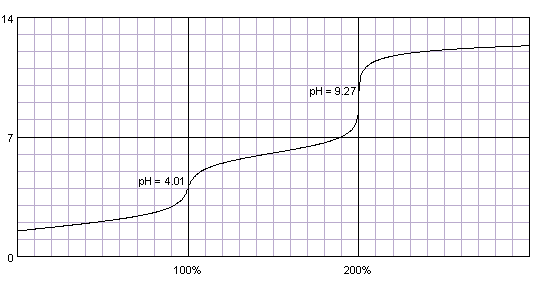# Predict the expected pH at the endpoint of the titration of weak acid and a strong base? Maleic Acid and NaOH?

Jul 3, 2017

The question is a bit ambiguous.........I would answer $\left(c\right)$, and I may be wrong........

#### Explanation:

Maleic acid reacts with $\text{TWO equiv}$ of sodium hydroxide......

${\text{HO"_2"CCH=CHCO"_2"H(aq)" + "2NaOH(aq)"rarr""^(-)"O"_2"CCH=CHCO"_2^(-)"Na}}_{2}^{+}$

Because it is a DIACID that requires 2 equiv of NaOH, at the endpoint we predict that we have a solution that is stoichiometric in ${\text{^(-)"O"_2"CCH=CHCO"_2^(-)"Na}}_{2}^{+}$. And we would expect this species to act as a WEAK base in water.......i.e.

$\text{^(-)"O"_2"CCH=CHCO"_2^(-)"Na"_2^+ + H_2O rightleftharpoons "HO"_2"CCH=CHCO"_2^(-)"Na"^+ + "NaOH(aq)}$

Are you following me? I know I am being a little bit abstract in suggesting that the diacid gets robbed of its two protons to form the dicarboxylate, and then itself robs the water of one proton to give equilibrium quantities of ${\text{HO"_2"CCH=CHCO"_2^(-)"Na}}^{+}$ (of course this association reaction will not be quantitative).

We could work out this reaction quantitatively, but I am going to have my breakfast first.......

Jul 3, 2017

1st equivalence point: (a) pH < 7 ; 2nd equivalence point: (c) pH > 7.

#### Explanation:

The question is worded ambiguously because maleic acid is a dibasic acid.

$\text{H"_2"A" + "H"_2"O" ⇌ "H"_3"O"^"+" + "HA"^"-"; "p"K_text(a1) = 1.9}$
$\text{HA"^"-" + "H"_2"O" ⇌ "H"_3"O"^"+" + "A"^"2-"; color(white)(ll)"p"K_text(a2) = 6.07}$

Thus, there are two equivalence points: one for the removal of the first proton and one for the second, similar to the titration curve below.pH at 1st equivalence point

A common formula for the pH at the first equivalence point of a dibasic acid is

color(blue)(bar(ul(|color(white)(a/a)"pH" = ("p"K_text(a1) + "p"K_text(a2))/2color(white)(a/a)|)))" "

$\text{pH} = \frac{1.9 + 6.07}{2} = 3.98$

pH at 2nd equivalence point

At this point, we have a solution of $\text{A"^"2-}$.

The equilibrium is

$\text{A"^"2-" + "H"_2"O" ⇌ "HA"^"-" + "OH"^"-}$

The conjugate base of a weak acid is a base, so the pH will be greater than 7, probably around pH 9.